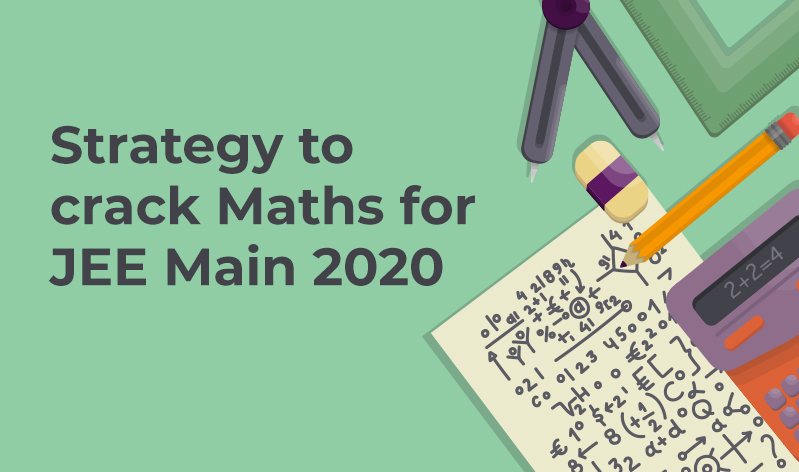# Preparation Strategy For JEE Main Mathematics 2020With hardly any time left for JEE Main 2020 January session, you must be going through the most anxious phase of your life now. Surrounded by books and notes, you must be feeling the heat, and that is understandable.

We also know that you face a lot of anxiousness when it comes to JEE Mathematics. All the equations and numbers always feel confusing for the brain. Well, we can help you sort out these woes to help you ace JEE Main 2020.

We have compiled some well-thought-out methods to deal with JEE Mathematics blues and help you overcome them. Keep on reading to know how to deal with JEE Maths in the shortest period:

Keep the pattern in mind: With the changes made in the exam pattern by JEE, it’s really important to keep the changes in mind while preparing. Remember that you now have to attempt just 25 questions, out of which, 5 will of numerical value questions. Also, they don’t carry any negative marking.

Know the weightage of each topic: You should always prepare while keeping in mind the weightage of each topic, especially at such a crucial stage. Here’s the topic-wise weightage:

• Sets, Relations and Functions – 5% weightage
• Complex numbers and quadratic equations – 7% weightage
• Matrices and Determinants – 7% weightage
• Permutations and combinations – 4% weightage
• Binomial theorem and its simple applications – 2% weightage
• Sequence and series – 5% weightage
• Integral Calculus – 9% weightage
• Limit, continuity and differentiability – 10% weightage
• Co-ordinate geometry – 15% weightage
• Three-Dimensional Geometry – 6% weightage
• Vector Algebra – 5% weightage
• Statistics and Probability – 8% weightage
• Trigonometry – 4% weightage
• Mathematical reasoning – 3% weightage
• Differential equations – 3% weightage
• Mathematical Induction – 3% weightage

Important chapters: It’s not a myth that knowing the important chapters of any subject helps a lot when it comes to the exam day. Following are the important chapters of JEE:

1. Limits, Continuity and Differentiability
2. Differentiation
3. Integration
4. Matrices and Determinants
5. Trigonometry
6. Sequence and Series
7. Probability
8. Sets and Functions
9. Statistics

## Tips To Crack Important Topics

Following are 7 handy tips to crack the important topics of Maths:

• In the case of Sequence and Series, in most of the cases, an arbitrary value helps. If the question is based on ‘n’, then put arbitrary values of ‘n’ and verify it. If the question is related to AM-GM, you can take the limiting values i.e. you can take all of them as equal.
• In the case of Coordinate Geometry, make a rough graph and mark the points or try the points given in option to satisfy the equation.
• The same method can be followed for Trigonometry. Suppose there are two variables A and B in a given question, try to put suitable known values (say A=60 and B=45) in question to reduce it to some constant, then put same values in given options one by one, whichever option matches the desired value would be the correct answer.
• Coordinate and Circles are also very important. Even for these chapters, you are supposed to remember lots of formulae and know when to apply which one.
• If there is partial marking, be very patient and attempt only as much as you know. Don’t guess, it can be fatal since -2 marking is there in multi correct.
• 3D Geometry, Vector and Integrals are high scoring and primarily calculation-based. Practise these chapters diligently to score full marks.
• Stats, Probability and PnC get the least weightage and are arguably the toughest portions. You need a good understanding of the concepts and a substantial amount of practise to crack the questions of these chapters.

## How To Solve Numerical Questions

You shouldn’t miss out on grabbing full 20 marks in the 5 numerical value questions. Also, there is no negative marking, so they should be definitely attempted. Following are some smart ways to solving them:

• Follow the basics of the question.
• Read them carefully, analyse and then solve.
• After completing the question, do recheck your calculations and then move ahead.

## Wind-up

Apart from all the above hacks and tips, there is one thing which is a must-do thing for you – that is to practice mock tests on a real NTA screen. You can identify your weakness and strengths through the mock tests and work on them accordingly.

You should also attempt previous year papers to practice more and get used to the type of questions that you might counter in the exam.

We wish you all the best!